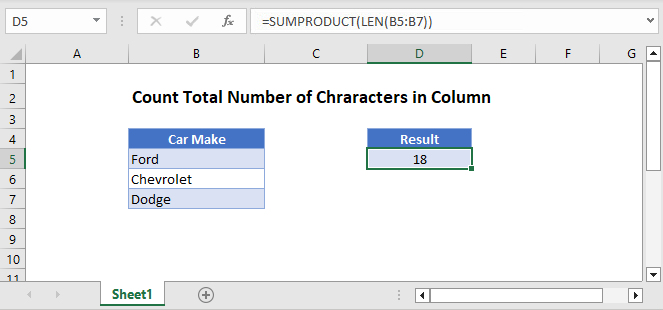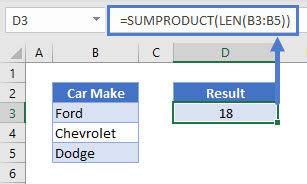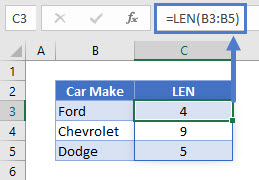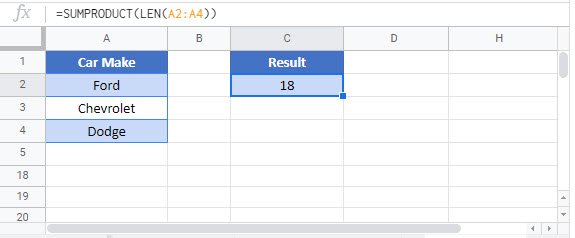# Count Total Number of Characters in Column – Excel & Google Sheets

Last updated on September 11, 2023

This tutorial will demonstrate how to count the total number of characters in a column in Excel and Google Sheets.## SUMPRODUCT and LEN Functions

To count the total number of characters in a column we can use the SUMPRODUCT and LEN Functions.

``=SUMPRODUCT(LEN(B3:B5))``## LEN Function

First, we can use the LEN function can be used to give us the length of the text in the cell range selected.

``=LEN(B3:B3)``## SUMPRODUCT Function

Next, we can use the SUMPRODUCT Function to show the number of characters in the column as a total.

``=SUMPRODUCT(LEN(B3:B5))``## Count Total Number of Characters in Column in Google Sheets

The example above works the same way in google sheets.### Excel Practice Worksheet

Practice Excel functions and formulas with our 100% free practice worksheets!